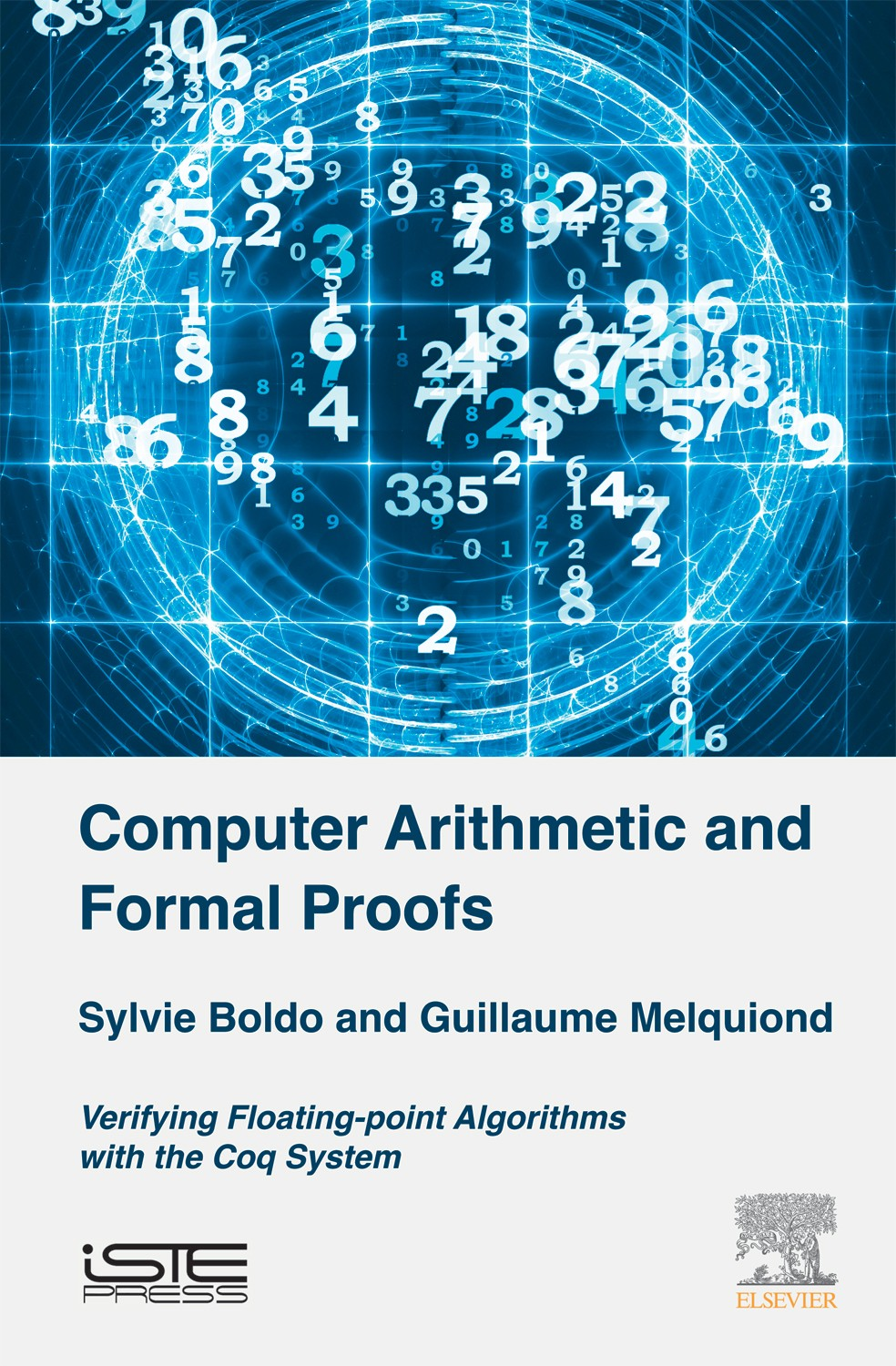pages
326
ISBN
9781785481123

Floating-point arithmetic is ubiquitous in modern computing, as it is the tool of choice to approximate real numbers. Due to its limited range and precision, its use can become quite involved and potentially lead to numerous failures. One way to greatly increase confidence in floating-point software is by computer-assisted verification of its correctness proofs. This […]

Disponible sur Amazon

Floating-point arithmetic is ubiquitous in modern computing, as it is the tool of choice to approximate real numbers. Due to its limited range and precision, its use can become quite involved and potentially lead to numerous failures. One way to greatly increase confidence in floating-point software is by computer-assisted verification of its correctness proofs.

This book provides a comprehensive view of how to formally specify and verify tricky floating-point algorithms with the Coq proof assistant. It describes the Flocq formalization of floating-point arithmetic and some methods to automate theorem proofs. It then presents the specification and verification of various algorithms, from error-free transformations to a numerical scheme for a partial differential equation. The examples cover not only mathematical algorithms but also C programs as well as issues related to compilation.

1. Floating-Point Arithmetic. 2. The Coq System. 3. Formalization of Formats and Basic Operators. 4. Automated Methods. 5. Error-Free Computations and Applications. 6. Example Proofs of Advanced Operators. 7. Compilation of FP Programs. 8. Deductive Program Verification. 9. Real and Numerical Analysis.

## Sylvie Boldo

Sylvie Boldo is a Research Director at Inria in Orsay, France.